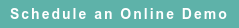# RoyaltyStat Blog

### Posts by Topic

It’s often stated that crude oil and natural gas (hereafter energy) prices are determined by supply and demand conditions. The empirical evidence shows that this notion is false because energy prices follow an autoregressive mechanism. Energy prices approach a random walk in which the autoregression slope coefficient is not different from one.

Here, I consider the daily Henry Hub Natural Gas Spot Prices (USD per million BTU) and daily Cushing, Oklahoma, WTI Spot Price FOB (USD per Barrel) using all the available data in U.S. Energy Information Administration (EIA). The (t) in parentheses in the equations below denotes time, such as day count.

Daily natural gas spot prices are explained by the autoregression equation:

##### (1) H(t) = 0.0618 + 0.9852 H(t – 1) + random errors

Gosset´s t-Statistics = 6.101 and 455.47
Standard error of regression = 0.3721 and R-Squared = 0.9706

The average H(t) = \$4.15 per million BTU, standard deviation = \$2.17, and count = 6,283 included daily observations from January 8, 1997 to December 31, 2021.

Data source: https://www.eia.gov/dnav/ng/hist/rngwhhdD.htm

Daily crude oil spot prices are explained by the autoregression equation:

##### (2) P(t) = 0.0565 + 0.9989 P(t – 1) + random errors

Gosset´s t-Statistics = 2.0851 and 1958.28
Standard error of regression = 1.3977 and R-Squared = 0.9976

The average P(t) = \$44.69 per Barrel, standard deviation = \$28.77, and count = 9,071 included daily observations from January 3, 1986 to December 31, 2021.

Data source: https://www.eia.gov/dnav/pet/hist/RWTCD.htm

To be tractable, I refrain from the statistical test of unit roots. As a statistical matter, both natural gas, H(t) and crude oil prices, P(t) are random walks around a drift coefficient.

Supply and demand conditions are false explanations of natural gas and crude oil prices. They may explain random shocks (large deviations from the central drift estimate); but supply and demand conditions do not explain the intrinsic (random walk) behavior of energy prices. Thus, government induced austerity (demand repression) to control prolonged price spikes is ill-informed policy.

#### Appendix: Autoregressive Model

The autoregression model can be interpreted as a constant value of the dependent variable plus a decaying weighted series of random shocks:

##### (3) Y(t) = α + β Y(t – 1) + U(t),

where U(t) is random uncertainty (errors or supply shocks such as sanctions). The expected value of U(t) = 0.

Alpha in equation (3) is the intercept and beta is the slope coefficient. I let

##### (4) Y(t – 1) = S Y(t),

where S is a time shift operator. I substitute (4) into (5), and obtain:

##### (8) Y(t) = α / (1 − µ) + U(t) / (1 − µ)

because I let the parameter µ = β S.

From the algebraic series truncation (see G. Dahlquist and A. Björck, equation 3.1.4, p. 66), 1 / (1 − µ) = 1 + µ + µ2 + µ3 + …

After I substitute this series truncation formula into equation (8), the price drift plus a weighted series of random shocks become visible:

##### (9) Y(t) = C + (1 + µ + µ2 + µ3 + …) U(t)

(10) Y(t) = C + U(t) + µ U(t) + µ2 U(t) + µ3 U(t)

The reduced-form intercept C = alpha / (one minus mu) is the constant (or fixed) value of the dependent variable covering the whole period. The slope coefficient in both H(t) and P(t) autoregressions is near one, implying that the deflator (one minus mu) is near zero, and the truncated weights of the random errors of equation (10) are explosive.

#### References

G. Dahlquist and A. Björck, Numerical Methods, Prentice-Hall, 1974. This well-written book is available in iBooks and Kindle.

Salvatore Carollo, Understanding Oil Prices, Wiley Finance, 2012, claims (pp. 3, 75) that crude oil price = ƒ(demand, supply), without specifying the supply and demand functions. He claims also (p. 31) that crude oil price = Benchmark price ± Differential, again without specifying the price differential.

The statistical unit root test is explained here (including additional references): https://en.m.wikipedia.org/wiki/Unit_root_test.

U.S. Energy Information Administration, "Energy & Financial Markets: What Drives Crude Oil Prices?" www.eia.gov/finance/markets/crudeoil.

Published on Aug 15, 2022 9:48:09 AM

Ednaldo Silva (Ph.D.) is founder and managing director of RoyaltyStat. He helped draft the US transfer pricing regulations and developed the comparable profits method called TNNM by the OECD. He can be contacted at: esilva@royaltystat.com

RoyaltyStat provides premier online databases of royalty rates extracted from unredacted license agreements
and normalized company financials (income statement, balance sheet, cash flow). We provide high-quality data, built-in analytical tools, customer training and attentive technical support.